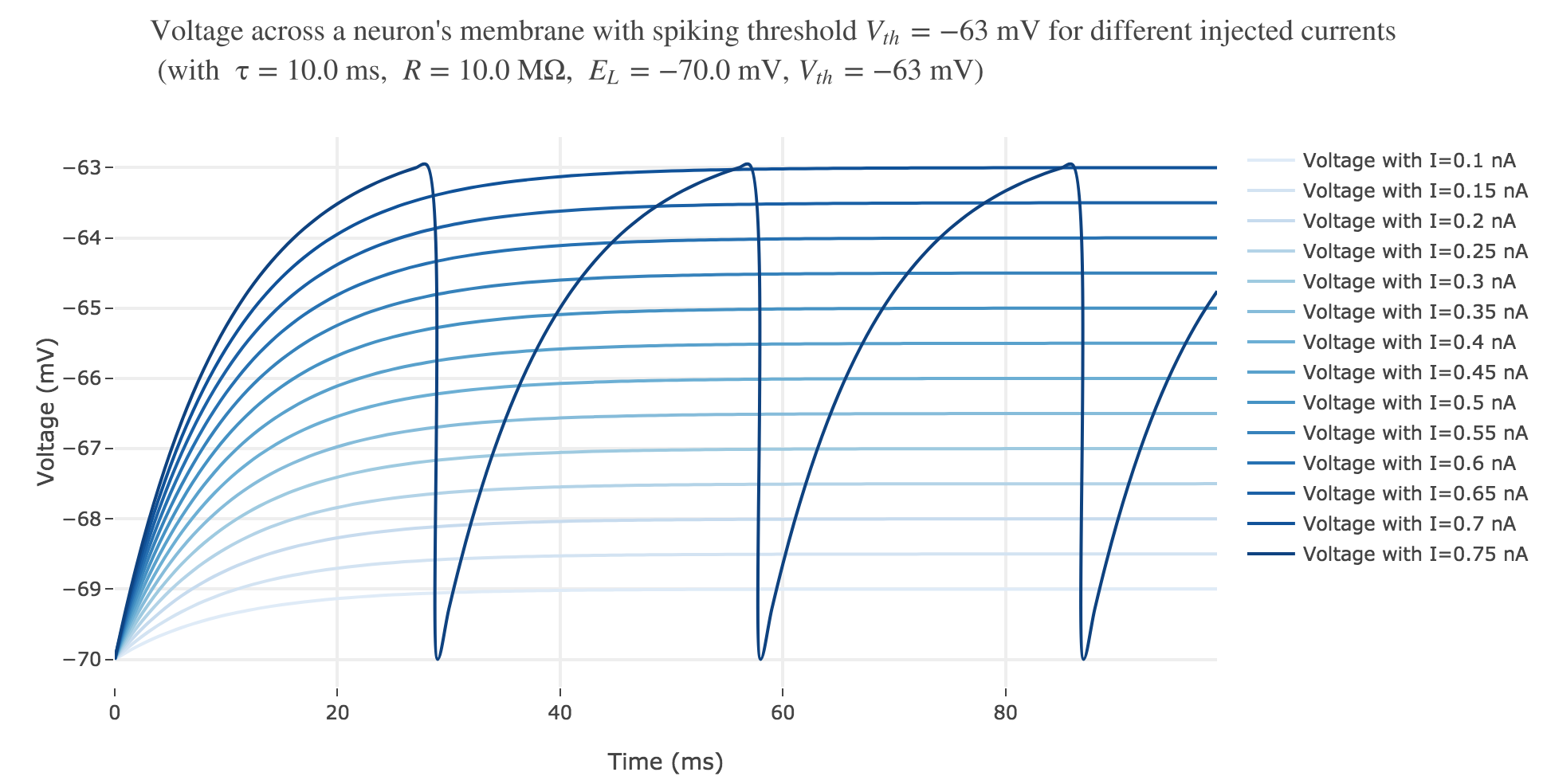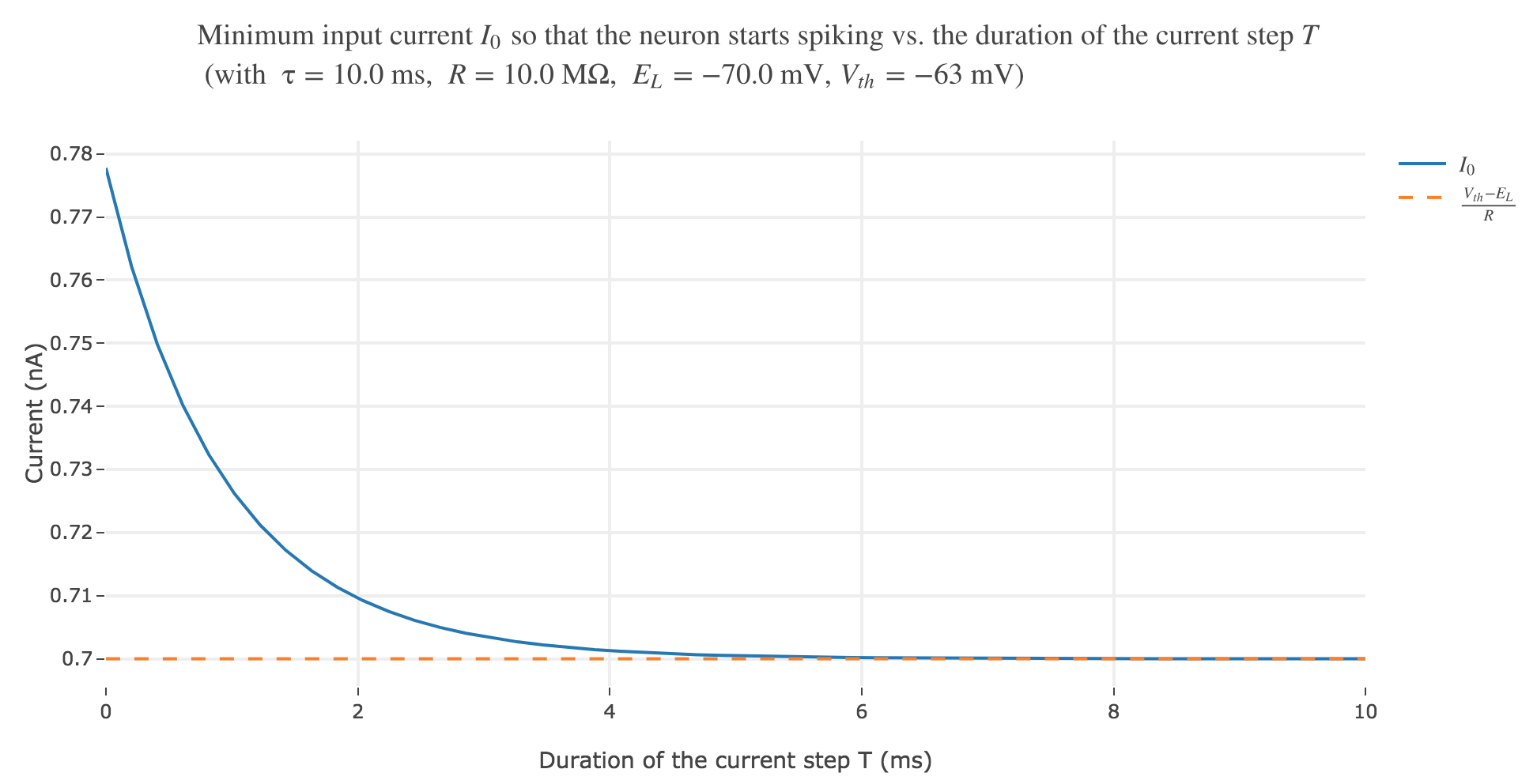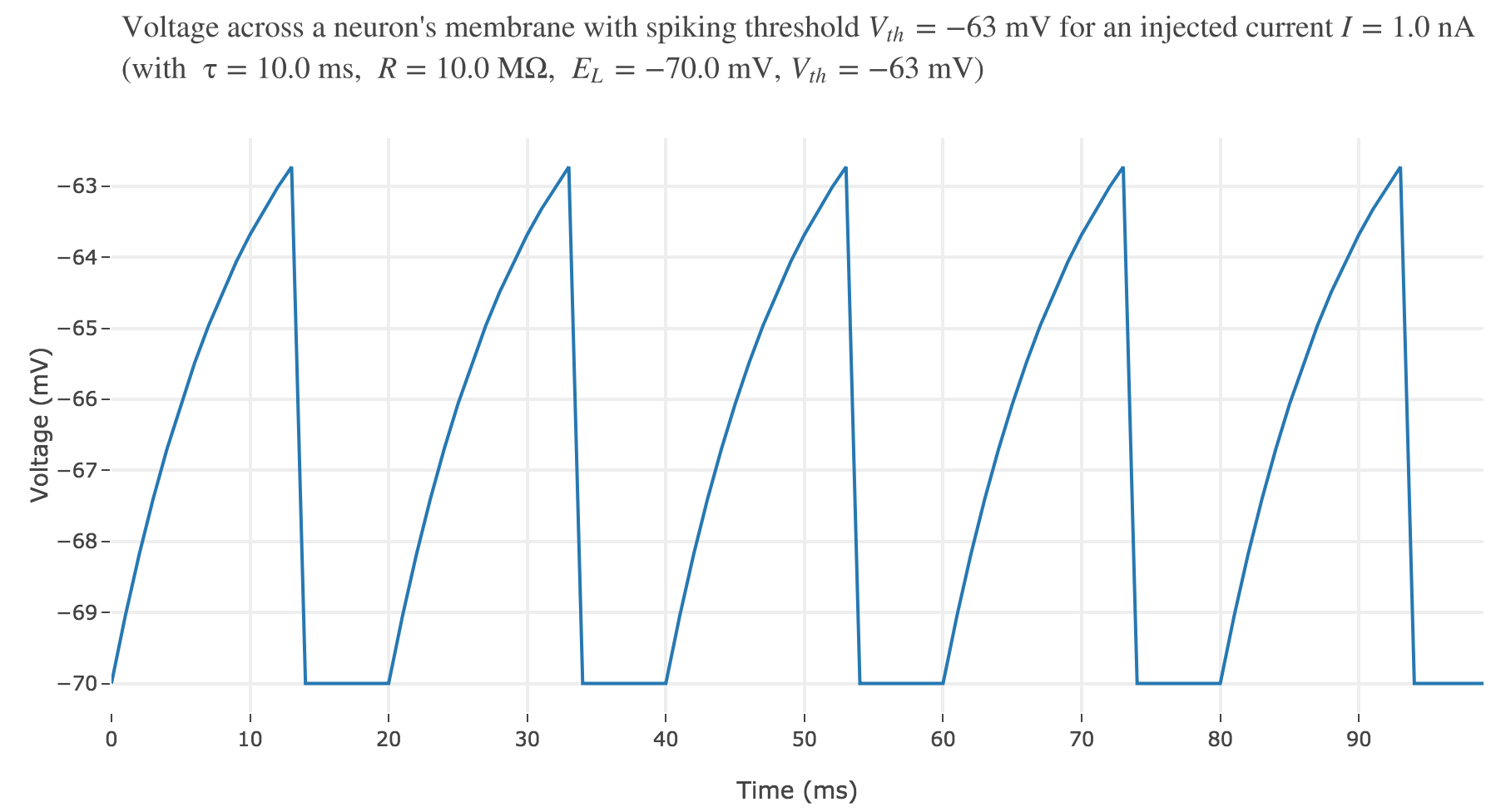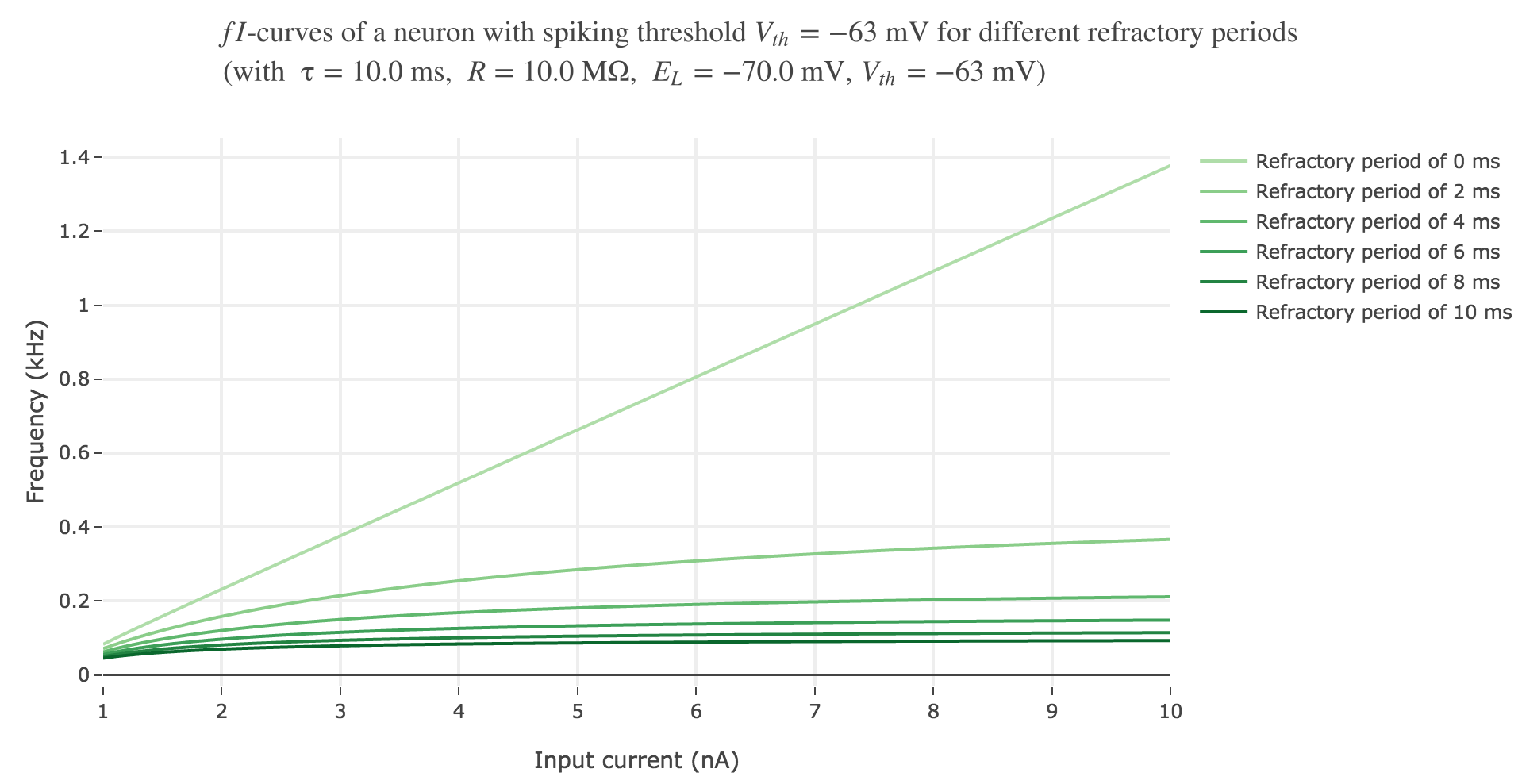# Exercise Sheet 6: Integrate-and-Fire Neuron

### Younesse Kaddar

\newcommand{\d}{ {\rm d}} \newcommand{\e}{ {\rm e}}

# 1. Integrate-and-Fire Neuron

We consider the integrate-and-fire neuron with differential equation:

τ \frac{\d V(t)}{\d t} = E_L - V(t) + RI(t)

Whenever the voltage reaches a threshold, $V ≥ V_{\text{th}}$ is set back to the equilibrium potential $V = E_L$

## a). Consider a constant current $I(t) = I_0$. How large must $I_0$ be so that the neuron starts spiking?

Let us solve the integrate-and-fire neuron’s differential equation in this case:

\begin{align*} \quad & τ \frac{\d V(t)}{\d t} = E_L - V(t) + RI_0\\ ⟺ \quad & \frac{ \d V(t)}{ \d t} = \frac{E_L + RI_0}{τ} - \frac{V(t)}{τ}\\ ⟺ \quad & \frac{ \d V(t)}{ \d t} + \frac 1 {τ} \, V(t) = \frac {E_L + RI_0} {τ}\\ ⟺ \quad & \frac{ \d V(t)}{ \d t} \, \e^{t/τ} + \frac 1 {τ} \, V(t) \, \e^{t/τ} = \frac {E_L + RI_0} {τ} \, \e^{t/τ}\\ ⟺ \quad & \frac{ \d}{ \d t} \left[V(t) \, \e^{t/τ}\right] = \frac {E_L + RI_0} {τ} \, \e^{t/τ}\\ ⟺ \quad & V(t) \, \e^{t/τ} =\frac {E_L + RI_0} {τ} \, τ \; \e^{t/τ} + \text{const}\\ ⟺ \quad & V(t) \, \e^{t/τ} = \left(E_L + R I_0 \right) \, \e^{t/τ} + \text{const}\\ ⟺ \quad & V(t) = \left(E_L + R I_0 \right) + \text{const} × \e^{-t/τ}\\ \end{align*}

And with $V(0) = E_L$:

E_L = E_L + R I_0 + \text{const} \; ⟺ \; \text{const} = - R I_0

Therefore:

V(t) \, = E_L + R I_0 \left(1-\e^{-t/τ}\right)

As a result:

\begin{align*} \quad & \; V(t) \, \overset{\text{eventually}}{≥} V_{th}\\ ⟺ \quad & \; \underbrace{\lim\limits_{t \to +∞} V(t)}_{= \; E_L + R I_0} ≥ V_{th} &&\text{(as } V \text{ is monotonous before spiking)}\\ ⟺ \quad & \; E_L + R I_0 ≥ V_{th}\\ ⟺ \quad & \; I_0 ≥ \frac{V_{th} - E_L}{R} \end{align*}

Thus, for the neuron to start spiking, it is necessary that:

I_0 ≥ \frac{V_{th} - E_L}{R}

This is illustrated by the following plot, where we set

• $R ≝ 10 \text{ MΩ}$
• $E_L ≝ −70 \text{ mV}$ (reversal potential)
• $τ ≝ 10 \text{ ms}$
• $V_{th} ≝ -63 \text{ mV}$

as a result of which $\frac{V_{th} - E_L}{R} = 0.7 \text{ nA}$:Figure 1.a. - Voltage accross a neuron's membrane with spiking threshold $V_{th} ≝ −63 \text{ mV}$ for different injected currents

## b). Let us now consider the case of a current step.

In this case, we turn on a current at time $t=t_0$ and then turn it off after $T$ time units. In other words, the current is given by:

I(t) = \begin{cases} 0 \text{ for } t<t_0 \\ I_0 \text{ for } t_0 ≤ t < t_0+T\\ 0 \text{ for } t ≥ t_0 + T \end{cases}

## How large does $I_0$ have to be, as a function of $T$, for the neuron to spike? Make a plot of $I_0$ versus $T$.

Analogously to what we did in the previous question, we can show that:

• for $t ∈ [0, t_0]$:

V(t) = E_L + \text{const} × \e^{-t/τ}

and since $V(0) = E_L$:

E_L = E_L + \text{const} \; ⟺ \; \text{const} = 0

that is:

V(t) = E_L
• for $t ∈ [t_0, t_0+T[$:

V(t) = E_L + RI_0 + \text{const} × \e^{-t/τ}

and since $V(t_0) = E_L$:

E_L = E_L + RI_0 + \text{const} × \e^{-t_0/τ} \; ⟺ \; \text{const} = - R I_0 \, \e^{t_0/τ}

that is:

V(t) \, = E_L + R I_0 \left(1-\e^{-(t-t_0)/τ}\right)
• for $t ∈ [t_0+T, +∞[$:

V(t) = E_L + \text{const} × \e^{-t/τ}

and since $V(t_0+T) = E_L + R I_0 \left(1-\e^{-T/τ}\right)$:

E_L + R I_0 \left(1-\e^{-T/τ}\right) = E_L + \text{const} × \e^{-(t_0+T)/τ} \; \\ ⟺ \; \text{const} = R I_0 \left(1 - \e^{-T/τ}\right) \e^{(t_0+T)/τ}

that is:

V(t) \, = E_L + R I_0 \left(1-\e^{-T/τ}\right) \, \e^{-(t-t_0-T)/τ}

Thus:

• for $t < t_0$, the membrane voltage is constant and equal to the equilibrium potential $E_L$

• for $t_0 ≤ t < t_0+T$, the voltage increases up to $V(t_0 + T) = E_L + R I_0 \left(1-\e^{-T/τ}\right)$

• for $t ≥ t_0 + T$, the voltage decreases to $\lim\limits_{t \to +∞} V(t) = E_L$

so the maximal value reached by $V$ is $V(t_0 + T) = E_L + R I_0 \left(1-\e^{-T/τ}\right)$

As a consequence, the neuron spikes if and only if:

\begin{align*} \quad & V(t_0 + T) = E_L + R I_0 \left(1-\e^{-T/τ}\right) ≥ V_{th} \\ ⟺ \quad & I_0 ≥ \frac{V_{th} - E_L}{R \left(1-\e^{-T/τ}\right)} \\ \end{align*}Figure 1.b. - Minimum input current $I_0$ so that the neuron starts spiking as a function of the duration of the current step (with the same parameters as in the previous question)

## c. Advanced: Imagine that two current steps of length $T$ are injected with a time delay of $Δt$. What is the maximum delay $Δt$ between the two steps until an action potential is emitted?

Let us assume that the first current step happens between $t_0$ and $t_0+T$ as before: then the second current step occurs between $t_0+T+Δt$ and $t_0+2T+Δt$ as they are delayed by $Δt$.

Similarly to what we did in the previous question:

• for $t ∈ [0, t_0]$:

V(t) = E_L
• for $t ∈ [t_0, t_0+T[$:

V(t) \, = E_L + R I_0 \left(1-\e^{-(t-t_0)/τ}\right)
• for $t ∈ [t_0+T, \, t_0 + T + Δt[$:

V(t) \, = E_L + R I_0 \left(1-\e^{-T/τ}\right) \, \e^{-(t-t_0-T)/τ}
• for $t ∈ [t_0+T+Δt, \, t_0 + 2T + Δt[$:

V(t) = E_L + RI_0 + \text{const} × \e^{-t/τ}

and since $V(t_0 + T + Δt) = E_L + R I_0 \left(1-\e^{-T/τ}\right) \, \e^{-Δt/τ}$:

E_L + R I_0 \left(1-\e^{-T/τ}\right) \, \e^{-Δt/τ} = E_L + RI_0 + \text{const} × \e^{-(t_0+T+Δt)/τ} \\ ⟺ \; \text{const} = R I_0 \; \e^{(t_0+T+Δt)/τ} \left(\big(1-\e^{-T/τ}\big)\, \e^{-Δt/τ} -1\right)

that is:

V(t) \, = E_L + R I_0 \Bigg(1 + \e^{-(t-t_0-T-Δt)/τ} \left(\big(1-\e^{-T/τ}\big)\, \e^{-Δt/τ} -1\right)\Bigg)
• for $t ∈ [t_0+2T+Δt, \, + ∞[$:

V(t) = E_L + \text{const} × \e^{-t/τ}

and since $V(t_0+2T+Δt) = E_L + R I_0 \left(1 + \e^{-T/τ} \Big(\left(1-\e^{-T/τ}\right)\, \e^{-Δt/τ} -1\Big)\right)$:

E_L + R I_0 \left(1 + \e^{-T/τ} \Big(\left(1-\e^{-T/τ}\right)\, \e^{-Δt/τ} -1\Big)\right) = E_L + \text{const} × \e^{-(t_0+2T+Δt)/τ} \; \\ ⟺ \; \text{const} = R I_0 \, \e^{(t_0+2T+Δt)/τ} \; \left(1 + \e^{-T/τ} \Big(\left(1-\e^{-T/τ}\right)\, \e^{-Δt/τ} -1\Big)\right)

that is:

V(t) \, = E_L + R I_0 \, \left(1 + \e^{-T/τ} \Big(\left(1-\e^{-T/τ}\right)\, \e^{-Δt/τ} -1\Big)\right) \, \e^{-(t-t_0-2T-Δt)/τ}

Thus:

• for $t < t_0$, the membrane voltage is constantly equal to the equilibrium potential $E_L$

• for $t_0 ≤ t < t_0+T$, the voltage increases up to $V(t_0 + T) = E_L + R I_0 \left(1-\e^{-T/τ}\right)$

• for $t_0 + T ≤ t < t_0 + T + Δt$, the voltage decreases down to $V(t_0 + T + Δt) = E_L + R I_0 \left(1-\e^{-T/τ}\right) \, \e^{-Δt/τ}$

• for $t_0 + T + Δt ≤ t < t_0 + 2T + Δt$, the voltage increases up to $V(t_0 + 2T + Δt) = E_L + R I_0 \left(1 + \e^{-T/τ} \Big(\left(1-\e^{-T/τ}\right)\, \e^{-Δt/τ} -1\Big)\right)$

• for $t ≥ t_0 + 2T + Δt$, the voltage decreases to $\lim\limits_{t \to +∞} V(t) = E_L$

As

V(t_0 + 2T + Δt) = V(t_0 + T) + R I_0 \, \e^{-T/τ} \left(1-\e^{-T/τ}\right)\, \e^{-Δt/τ} > V(t_0 + T)

the maximal value reached by $V$ is $V(t_0 + 2T + Δt)$.

As a consequence, the neuron spikes if and only if:

\begin{align*} \quad & V(t_0 + 2T + Δt) ≥ V_{th} \\ ⟺ \quad & E_L + R I_0 \left(1 + \e^{-T/τ} \Big(\left(1-\e^{-T/τ}\right)\, \e^{-Δt/τ} -1\Big)\right) ≥ V_{th}\\ ⟺ \quad & \e^{-T/τ} \Big(\left(1-\e^{-T/τ}\right)\, \e^{-Δt/τ} -1\Big) ≥ \frac{V_{th} - E_L}{R I_0} - 1\\ ⟺ \quad & \left(1-\e^{-T/τ}\right)\, \e^{-Δt/τ} ≥ \e^{T/τ} \, \left(\frac{V_{th} - E_L}{R I_0} - 1\right)+1\\ ⟺ \quad & Δt ≤ -τ \; \ln \frac{\e^{T/τ} \, \left(\frac{V_{th} - E_L}{R I_0} - 1\right)+1}{1-\e^{-T/τ}}\\ \end{align*}

# 2. Integrate-and-Fire with refractory period

Real neurons usually have refractory periods, i.e. for a few milliseconds after an action potential, the neuron will not fire again.

## How could you add such a refractory period to the integrate-and-fire neuron?

To add a refractory period $Δ$, we make the voltage stay constant at the equilibirum potential $E_L$ for a time span $Δ$ whenever it is reset to $E_L$ after reaching the threshold.Figure 2.a.1. - Voltage across a neuron's membrane with spiking threshold $V_{th} = -63 \text{ mV}$ and a $5 \text{ ms}$ refractory period

## Compute the new $fI$-curve, i.e. the firing rate versus input current curve of the neuron with refractory period. How does it differ from the old one?

Let us denote by $T$ the time to threshold when there is no refractory period:

\begin{align*} \quad & τ \frac{\d V(t)}{\d t} = E_L - V(t) + RI(t) \quad \text{ and } \quad V(0) = E_L \\ ⟺ \quad & V(t) \, = E_L + R I_0 \left(1-\e^{-t/τ}\right) \\ \end{align*}

and by definition of $T$:

\begin{align*} \quad & V(T) = V_{th} \\ ⟺ \quad & E_L + R I_0 \left(1-\e^{-T/τ}\right) = V_{th} \\ ⟺ \quad & \e^{-T/τ} = 1- \frac{V_{th} - E_L}{RI_0} \\ ⟺ \quad & T = - τ \, \ln\left(1- \frac{V_{th} - E_L}{RI_0}\right) \\ ⟺ \quad & T = τ \, \ln\left(\frac{RI_0}{RI_0 - V_{th} + E_L}\right) \\ \end{align*}

and the firing rate $f$ is equal to:

f ≝ \frac 1 T = \left(τ \, \ln\left(\frac{RI_0}{RI_0 - V_{th} + E_L}\right)\right)^{-1}

So when there is a refractory period $Δ$:

T_{ref} = τ \, \ln\left(\frac{RI_0}{RI_0 - V_{th} + E_L}\right) + Δ

as a result of which the firing rate is equal to:

f_{ref} ≝ \frac {1} {T_{ref}} = \left(τ \, \ln\left(\frac{RI_0}{RI_0 - V_{th} + E_L}\right) + Δ\right)^{-1}

Therefore, compared to $f$:

• the firing rate $f_{ref}$ is now bounded by $\frac{1}{Δ}$:

f_{ref} = \Bigg(\underbrace{τ \, \ln\left(\frac{RI_0}{RI_0 - V_{th} + E_L}\right)}_{≥ 0} + Δ\Bigg)^{-1} ≤ \frac{1}{Δ}
• and it is lower than the firing rate without refractory period $f$:

f_{ref} = \Bigg(τ \, \ln\left(\frac{RI_0}{RI_0 - V_{th} + E_L}\right) + \underbrace{Δ}_{≥ 0}\Bigg)^{-1} ≤ \frac{1}{τ \, \ln\left(\frac{RI_0}{RI_0 - V_{th} + E_L}\right)} = fFigure 2.a.2. - $fI$-curves of a neuron with spiking threshold $V_{th} = -63 \text{ mV}$ for different refractory periods

Tags:

Updated: